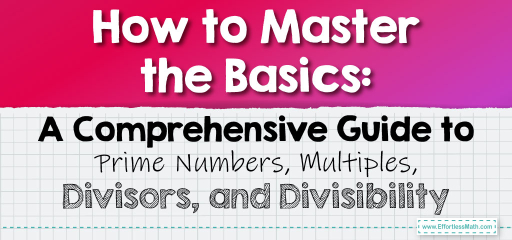# How to Master the Basics: A Comprehensive Guide to Prime Numbers, Multiples, Divisors, and Divisibility

Understanding primes, prime numbers, multiples, divisors, and divisibility involves several fundamental concepts in mathematics. Let's break down each concept step-by-step for a clear understanding.## Step-by-step Guide to Master Prime Numbers, Multiples, Divisors, and Divisibility

### 1. Prime Numbers

• Definition: A prime number is a natural number greater than $$1$$ that has no positive divisors other than $$1$$ and itself. In other words, it cannot be formed by multiplying two smaller natural numbers.
• Examples: $$2, 3, 5, 7, 11, 13$$, etc.
• Identifying Prime Numbers:
• Check if a number greater than $$1$$ is divisible only by $$1$$ and itself.
• Use methods like trial division, where you try dividing the number by all natural numbers up to its square root.

### 2. Understanding Multiples

• Definition: A multiple of a number is the product of that number and an integer.
• Examples:
• Multiples of $$2$$: $$4, 6, 8, 10, 12$$, etc.
• Multiples of $$5$$: $$10, 15, 20, 25$$, etc.
• Identifying Multiples:
• Multiply the number by various integers (positive or negative) to find its multiples.

### 3. Divisors

• Definition: A divisor of a number is an integer that can be multiplied by another integer to produce the number.
• Examples:
• Divisors of $$6$$: $$1, 2, 3, 6$$.
• Divisors of $$15$$: $$1, 3, 5, 15$$.
• Identifying Divisors:
• Find all numbers that divide the given number without leaving a remainder.

### 4. Divisibility

• Definition: A number is divisible by another if, upon division, the result is an integer with no remainder.
• Divisibility Rules:
• For $$2$$: A number is divisible by $$2$$ if its last digit is even.
• For $$3$$: A number is divisible by $$3$$ if the sum of its digits is divisible by $$3$$.
• For $$5$$: A number is divisible by $$5$$ if its last digit is $$0$$ or $$5$$.
• Applying Divisibility:
• Use divisibility rules to quickly determine if one number can be divided evenly by another.

### 5. Practice and Application

• Problem Solving: Apply these concepts to solve problems in number theory, algebra, and real-world scenarios.
• Practical Examples: Determine if a number is prime, find all divisors of a given number, or use divisibility rules to simplify calculations.

• Explore concepts like prime factorization, greatest common divisors, least common multiples, etc., for a deeper understanding.

## Final Word

• Prime numbers are foundational in mathematics, and understanding their properties, along with concepts of multiples, divisors, and divisibility, is crucial in various mathematical fields.
• Regular practice and application of these concepts help in strengthening mathematical proficiency and problem-solving skills.

### Examples:

Example 1:

Is $$17$$ a prime number?

Solution:

• A prime number is a natural number greater than $$1$$ that has no positive divisors other than $$1$$ and itself.
• To determine if $$17$$ is a prime number, check if it has any divisors other than $$1$$ and $$17$$.
• Since $$17$$ cannot be divided evenly by any number other than $$1$$ and $$17$$, it is a prime number.

Example 2:

What are the divisors of $$12$$?

Solution:

• Divisors of a number are numbers that divide it evenly.
• The divisors of $$12$$ are $$1, 2, 3, 4, 6$$, and $$12$$ since:
• $$12÷1=12$$
• $$12÷2=6$$
• $$12÷3=4$$
• $$12÷4=3$$
• $$12÷6=2$$
• $$12÷12=1$$

### What people say about "How to Master the Basics: A Comprehensive Guide to Prime Numbers, Multiples, Divisors, and Divisibility - Effortless Math: We Help Students Learn to LOVE Mathematics"?

No one replied yet.

X
30% OFF

Limited time only!

Save Over 30%

SAVE $5 It was$16.99 now it is \$11.99